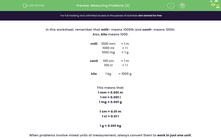# Measuring Problems (2)

In this worksheet, students will solve problems involving the calculation and conversion of units of measure, using decimal notation up to three decimal places.Key stage:  KS 2

Curriculum topic:   Measurement

Curriculum subtopic:   Solve Conversion Problems

Difficulty level:#### Worksheet Overview

In this worksheet, remember that milli- means 1000th and centi- means 100th.

Also, kilo means 1000.

 milli 1000 mm = 1 m 1000 ml = 1 l 1000 mg = 1 g centi 100 cm = 1 m 100 cl = 1 l kilo 1 kg = 1000 g

This means that:

1 mm = 0.001 m

1 ml = 0.001 l

1 mg = 0.001 g

1 cm = 0.01 m

1 cl = 0.01 l

1 g = 0.001 kg

When problems involve mixed units of measurement, always convert them to work in just one unit.

Often it is easier to work with the smaller unit of measurement and then convert back at the end if the question asks for the answer in the larger unit of measurement.

Example 1

Jack pours out 2 litres of water into an empty pan and then removes 132 ml. He then adds another 50 cl.

How much is now in the pan in litres?

Work in millilitres.

2000 ml - 132 ml + 500 ml = 2368 ml

Convert back to litres by dividing by 1000.

2.368 l is in the pan.

Example 2

Ann joins a 52 cm pipe, end to end, to one that is 2.5 m long.

She then realises that this is too long and removes 52 mm of pipe.

How long is the pipe now in m?

Work in cm.

52 cm + 250 cm - 5.2 cm = 296.8 cm

Convert back to m by dividing by 100.

The pipe is 2.968 m long.

### What is EdPlace?

We're your National Curriculum aligned online education content provider helping each child succeed in English, maths and science from year 1 to GCSE. With an EdPlace account you’ll be able to track and measure progress, helping each child achieve their best. We build confidence and attainment by personalising each child’s learning at a level that suits them.

Get started